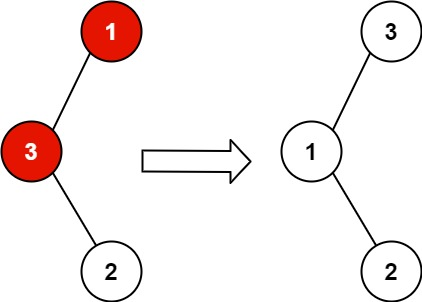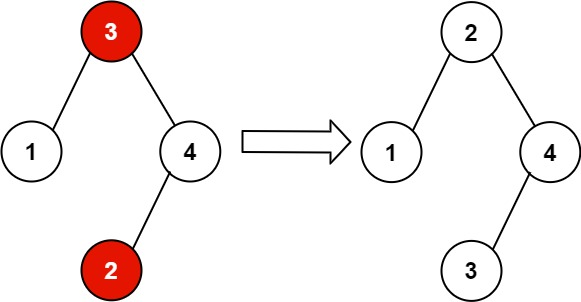### LeetCode• ㊗️
• 大家
• offer
• 多多！

## Problem

You are given the root of a binary search tree (BST), where exactly two nodes of the tree were swapped by mistake. Recover the tree without changing its structure.

Example 1:Input: root = [1,3,null,null,2]
Output: [3,1,null,null,2]
Explanation: 3 cannot be a left child of 1 because 3 > 1. Swapping 1 and 3 makes the BST valid.


Example 2:Input: root = [3,1,4,null,null,2]
Output: [2,1,4,null,null,3]
Explanation: 2 cannot be in the right subtree of 3 because 2 < 3. Swapping 2 and 3 makes the BST valid.


Constraints:

• The number of nodes in the tree is in the range $[2, 1000]$.
• $-2^{31} <= Node.val <= 2^{31} - 1$

Follow up: A solution using O(n) space is pretty straight-forward. Could you devise a constant O(1) space solution?

## Code

recursion

class Solution {
// 第一个错误值
TreeNode first = null;
// 第二个错误值
TreeNode second = null;
// 之前的节点
TreeNode prev = null;
public void recoverTree(TreeNode root) {
if(root == null) return;

// 找到first和second
helper(root);

int temp = first.val;
first.val = second.val;
second.val = temp;
}
// 中序遍历
private void helper(TreeNode root){
if(root == null) return;

helper(root.left);
// 出现了错误的值，中序遍历之前的值比之后的值大
if(prev != null && prev.val >= root.val){
// 有两个数顺序不对
// 第一个错误是prev
// 第二个错误是root
// [1,(3),(2),4,5]
// [1,(4),3,(2),5]
if(first == null) first = prev;
second = root;
}
prev = root;
helper(root.right);
}
}


stack

class Solution {
public void recoverTree(TreeNode root) {
if(root == null) return;
TreeNode first = null;
TreeNode second = null;
TreeNode prev = null;

TreeNode curr = root;
Stack<TreeNode> stack = new Stack<>();
while(!stack.isEmpty() || curr != null){
if(curr != null){
stack.push(curr);
curr = curr.left;
} else{
curr = stack.pop();
if(prev != null && prev.val >= curr.val){
if(first == null) first = prev;
second = curr;
}

prev = curr;
curr = curr.right;
}
}

int temp = first.val;
first.val = second.val;
second.val = temp;
}
}